Common Core: 8th Grade Math : Compare the Size of Irrational Numbers and Place them on a Number Line: CCSS.Math.Content.8.NS.A.2

Example Questions

← Previous 1

Example Question #1 : Compare The Size Of Irrational Numbers And Place Them On A Number Line: Ccss.Math.Content.8.Ns.A.2

Which point on the number line best represents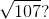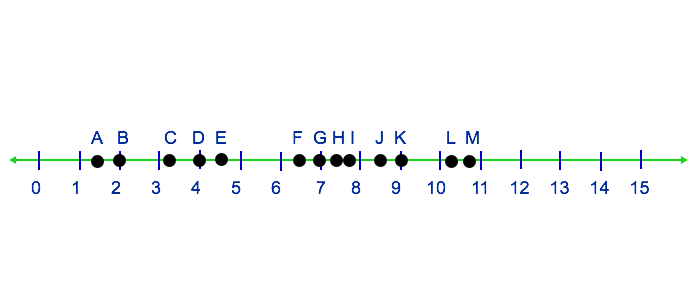K

M

L

J

L

Explanation:

To solve this problem, we can think of perfect squares around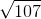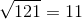This means thathas to be betweenandon the number line, but closer tobecause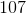is closer tothan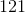. Based on the number line provided, point L is the best representation ofExample Question #1 : Compare The Size Of Irrational Numbers And Place Them On A Number Line: Ccss.Math.Content.8.Ns.A.2

Which point on the number line best represents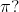A

D

C

B

C

Explanation:

To solve this problem, we first need to know what number the symbolrepresents: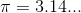We are looking for a point on the number line that is betweenand, but much closer to. Based on the number line provided, point C is the best representation ofExample Question #2 : Compare The Size Of Irrational Numbers And Place Them On A Number Line: Ccss.Math.Content.8.Ns.A.2

Which point on the number line best represents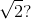C

D

B

A

A

Explanation:

To solve this problem, we first need to know what numberrepresents: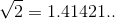We are looking for a point on the number line that is betweenand, about right between them. Based on the number line provided, point A is the best representation ofExample Question #1 : Compare The Size Of Irrational Numbers And Place Them On A Number Line: Ccss.Math.Content.8.Ns.A.2

Which point on the number line best represents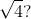B

A

D

C

B

Explanation:

To solve this problem, we first need to know what number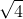represents:We are looking for a point on the number line that is on. Based on the number line provided, point B is the best representation ofExample Question #2 : Compare The Size Of Irrational Numbers And Place Them On A Number Line: Ccss.Math.Content.8.Ns.A.2

Which point on the number line best represents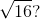B

C

A

D

D

Explanation:

To solve this problem, we first need to know what number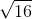represents:We are looking for a point on the number line that is on. Based on the number line provided, point D is the best representation ofExample Question #22 : The Number System

Which point on the number line best represents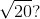G

H

F

E

E

Explanation:

To solve this problem, we can think of perfect squares around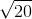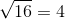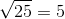This means thathas to be betweenandon the number line. Based on the number line provided, point E is the best representation ofExample Question #1 : Compare The Size Of Irrational Numbers And Place Them On A Number Line: Ccss.Math.Content.8.Ns.A.2

Which point on the number line best represents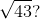F

H

G

E

F

Explanation:

To solve this problem, we can think of perfect squares around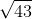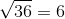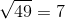This means thathas to be betweenandon the number line. Based on the number line provided, point F is the best representation ofExample Question #3 : Compare The Size Of Irrational Numbers And Place Them On A Number Line: Ccss.Math.Content.8.Ns.A.2

Which point on the number line best represents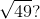F

H

E

G

G

Explanation:

To solve this problem, we first need to know what number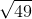represents:We are looking for a point on the number line that is on. Based on the number line provided, point G is the best representation ofExample Question #2 : Compare The Size Of Irrational Numbers And Place Them On A Number Line: Ccss.Math.Content.8.Ns.A.2

Which point on the number line best represents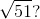E

G

H

F

H

Explanation:

To solve this problem, we can think of perfect squares around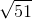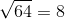This means thathas to be betweenandon the number line, but closer tobecause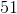is closer tothan. Based on the number line provided, point H is the best representation ofExample Question #1 : Compare The Size Of Irrational Numbers And Place Them On A Number Line: Ccss.Math.Content.8.Ns.A.2

Which point on the number line best represents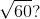I

K

J

L

I

Explanation:

To solve this problem, we can think of perfect squares around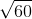This means thathas to be betweenandon the number line, but closer tobecause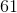is closer tothan. Based on the number line provided, point I is the best representation of← Previous 1

All Common Core: 8th Grade Math Resources